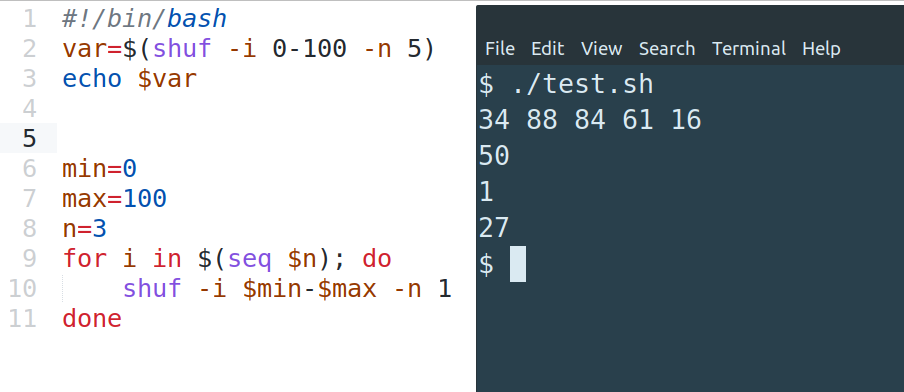To generate random number in range in bash or shell scripts we can use the `shuf` command.

(1) shuf - random numbers inclusive range

``````shuf -i 0-100 -n 2
``````

result:

``````55
69
``````

where:

• `-i` - defines the inclusive range: min and max
• `-n` - number of the random numbers to be produced

(2) RANDOM numbers from range

``````ceil=10
floor=1
echo \$(((RANDOM % \$((\$ceil- \$floor))) + \$floor))
``````

to run it as script don't set `#!/bin/bash` and run it by `./test.sh`. To learn more about shell scripts check: How to Write Script in Bash?

(3) assign random number to variable

``````#!/bin/bash
var=\$(shuf -i 0-100 -n 1)
echo \$var
``````

(4) append random number to string

``````var=\$(shuf -i 0-100 -n 1)
text="Hello "
echo "\${text}\${var}"
``````

result:

``````Hello 47
``````

(5) generate N random numbers from range

``````min=0
max=100
n=3
for i in \$(seq \$n); do
shuf -i \$min-\$max -n 1
done
``````

result:

``````65
29
70
``````

(6) bash generate random N digit number

random 6 digit number

``````((RND=RANDOM<<15|RANDOM)) ; echo \${RND: -6}
``````

random 8 digit number

``````((RND=RANDOM<<15|RANDOM)) ; echo \${RND: -8}
``````

Note: that some numbers might start with 0 - `05838933`

In this post we answer on those questions:

• bash random number between 1 and 100
• bash random range
• bash random number between 0 and 1
• bash random number fixed length
• bash generate random 8 digit number